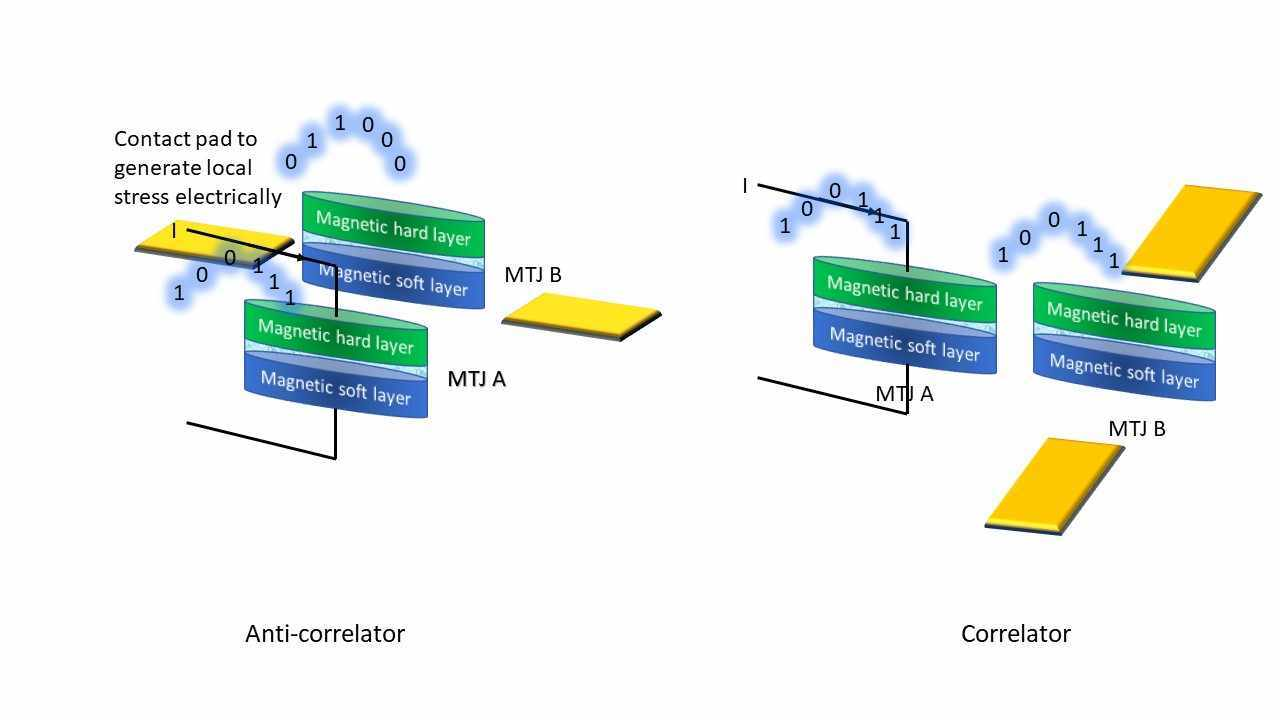# Electrically programmable probabilistic bit anti-correlator on a nanomagnetic platformIt is well-known that certain computational problems such as factorizing large numbers into their prime constituents, which is at the heart of many cryptography schemes, can be solved very efficiently with quantum computing. Classical computing is inefficient at such tasks, while probabilistic computing, a newer arrival, is more efficient than classical computing, but not as efficient as quantum computing.

Quantum computing, however, requires vast hardware resources which only the few and the fortunate can muster. Probabilistic computing, on the other hand, works with probabilistic (p-) bits, which are sometimes called “poor person’s quantum bits (qubits)”. P-bit based computing would presumably require less resources than quantum computing, but it turns out that the resource demand is actually much more than expected. This is primarily because probabilistic computing requires generating arbitrary correlations between p-bit streams which requires complex hardware like microcontrollers, shift registers, logic gates, etc. which have large footprint on a chip and consume excessive amount of energy.

In our work, we came up with a strategy to overcome this challenge. We showed that using two closely spaced magnetic devices known as magnetic tunnel junctions (MTJ), we can generate two p-bit streams with controllable correlations between them. The correlations are generated because of magnetic dipole interactions between the MTJs and the degree of correlation can be varied by subjecting one of the MTJs to electrically generated stress which modulates the effect of the dipole interaction influence it feels from the other MTJ. This paradigm replaces all the microcontrollers and shift registers, etc. with essentially no hardware at all since dipole coupling is a “wireless” interaction that consumes no area on a chip, and, by itself, dissipates no energy. Controlling the correlations with electrically generated stress however requires some energy, but it is several orders of magnitude smaller than what traditional hardware would require. This can make probabilistic computing both efficient and resource-frugal.

The above figure shows how to generate correlated or anti-correlated p-bit streams with two dipole- coupled MTJs. Depending on the relative placements of the two MYTJs, either correlation or anti-correlation is generated. The resistance state of either MTJ can be high or low, encoding bits 0 and 1. The probability with which the bit encoded in MTJ A is ‘1’ is controlled by the current I passing through it. The bit state of MTJ B is set by dipole interaction with MTJ A and that correlates or anti-correlates the two bits. The degree of correlation or anti-correlation is varied by subjecting MTJ B to varying amount of stress that is generated electrically by applying voltages to the contact pads. The structures are fabricated on a piezoelectric film (not shown) which is used to generate the stress.

This system can also act as a conditional probability generator or a simple 2-node Bayesian network. Bayesian networks are used to compute in the presence of uncertainty, such as stock market behavior, disease progression, etc. What makes this approach to implementing such systems attractive is the extremely small system footprint and the miniscule energy dissipation.

Commonwealth Professor

Virginia Commonwealth